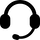# Online Partial differential equations tutor jobs

• ### Online Engineering Math, Partial differential equations tutor required in Vadodara

I am looking for a tutor for partial differential equations. This is a third year engineering math course to understand the level of difficulty. I will need help on June 22.

• 17 hours ago
• Vadodara, Gujarat, India
• \$20 (Fixed)
• ### Online Numerical Analysis, Partial differential equations tutor required in Irbid

I need expert in both: Numerical analysis at all levels, Partial differential equations also at all levels, I want to be expert in both

• Jun 18
• Irbid, Jordan
• \$28/hour
• ### Home | online Math tutor needed in Santiago de Querétaro

I want someone with a Ph.D. in analysis like Topolgy-Functional Analysis...... and also in a differential geometry subject

• 9 hours ago
• Santiago de Querétaro, Qro., Mexico
• \$2/hour
• ### Online Partial differential equations assignment help teacher needed in Lucknow

Complex variable and partial differential equations

•Jun 15
• Jun 14
• Lucknow, Uttar Pradesh, India
• ### Online Partial differential equations assignment help tutor required in Zahran

I am looking for someone have experience in partial

• Jun 12
• Abdoun Cir., Amman, Jordan
• \$56/hour
• ### Online Partial differential equations assignment help teacher required in Irbid

ا lam looking for someone with experience in ( Partial differential equations ) to solve my exam

• Jun 12
• Irbid, Jordan
• ### Online Geometry assignment help teacher needed in Irbid

I need an expert in each of the following: number theory, Euclid geometry, abstract algebra 1 and 2, real analysis 1 and 2, topological 1 and 2, partial differential equations, and finally numerical analysis, and please contact me

• Jun 12
• Irbid, Jordan
• \$31/hour
• ### Online Transforms and partial differential equations assignment help tutor required in Al Jubeiha

I need help in Partial Differential Equations task today please contact me as soon as possible.

• Jun 11
• Al Jubeiha, Amman, Jordan
• \$28 (Fixed)
• ### Online Partial differential equations assignment help teacher needed in Wadi As-Seir

I'm looking for someone who is expert in partial Differential Equation to help me in my test

• Jun 11
• Wadi As-Seir, Amman, Jordan
• \$28 (Fixed)
• ### Online Z-transform assignment help tutor needed in Kadapa

I need help for my maths exam

• Jun 11
• Jun 10
• Kadapa, Andhra Pradesh, India
• \$4/hour
• ### Home | online Engineering Maths tutor required in Teynampet

Need a tutor to help me understand some concepts and clear some doubts for engineering maths.mainly PDE and wave heat laplace equations
the topics i need to cover are
Multivariable calculus
Surface parametrization
surface integrals
integral theorems
ode
pde
periodic functions and fourier series
the laplace equation
heat equation...

• Jun 11
• Teynampet, Chennai, Tamil Nadu, India
• ### Online Renewable Energy Sources assignment help teacher needed in Lucknow

Renewable energy sources
Complex variable and partial differential equations
Material engineering technology
Matching process and metrology
I am looking for an online tutor for exams.

• Jun 10
• Jun 10
• Lucknow, Uttar Pradesh, India
• ### Online Transforms and partial differential equations assignment help teacher needed in Al Abdali

I'm looking for an expert in Partial differential equations
for more details
please contact me as soon as possible

• Jun 9
• Jabal Al Hussein, Amman, Jordan
• \$30/hour
• ### Online Partial differential equations assignment help teacher needed in Salem

should be available online at that particular hour and well versed in complex variables and partial diff

• Jun 9
• Salem, Tamil Nadu, India
• \$14/hour
• ### Online Partial differential equations assignment help tutor required in Vizianagaram

I am looking for a well experienced tutor to help me in my exams
Sub
1. APPLIED NUMERICAL METHODS
2.COMPLEX VARIABLES AND PARTIAL DIFFERENTIAL EQUATIONS
you can feel free to message (whatsapp)me if u know any of the above subjects. I am ready to pay

• Jun 9
• Vizianagaram, Andhra Pradesh, India
• \$7/hour
• ### Online Numerical Analysis assignment help tutor needed in SS 2

I am looking for an expert in numerical analysis. Someone who is very well versed with topics such as, taylor series, lagrange polynomial, neville's method, and more.

Newton-Cotes, trap and simpsons rule, recursive trapezoidal rule, richardson extrapolations and more... including gaussian quadrature, monte carlo method and multi-dimensional...

• Jun 9
• SS 2, Petaling Jaya, Selangor, Malaysia
• \$30 (Fixed)
• ### Online Differential Equations assignment help tutor needed in Çanakkale

I am looking for someone expert in differential equations in university level;
- First Order Differential Equations
- Second Order Differential Equations
- Higher Order Differential Equations
- Series Solutions of Second Order Linear Equations
- The Laplace Transform
- Systems of First Order Linear Equations
- Partial Differential Equations...

• Jun 8
• Çanakkale, Çanakkale Merkez/Çanakkale, Turkey
• \$18 (Fixed)
• ### Online Partial differential equations assignment help tutor needed in Irbid

Partial differential equations

• Jun 6
• Irbid, Jordan
• \$21/hour
• ### Online Partial differential equations assignment help tutor needed in Zarqa

I need professional guy to solve partial equations exam one hour next Thursday 35 dollar

• Jun 6
• Zarqa, Jordan
• \$35/hour
• ### Online Differential Equations assignment help tutor needed in Washington

i need help in Differential Equations with Boundary-Value course that im taking

• Jun 2
• Washington, DC, USA